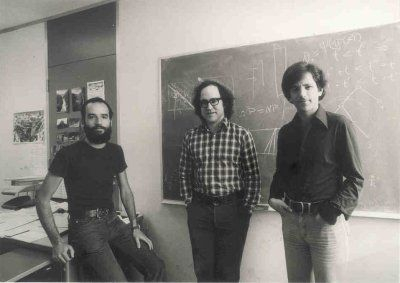## The RSA CryptosystemIntroduction     In 1976, Whitfield Diffie and Martin Hellman introduced to the world the concept of public key cryptography also known as asymmetric cryptography. But it wasn’t till 1977 when three people Ronald Rivest, Adi Shamir and Leonard Adleman proposed the scheme which would become the most used public key algorithm today called RSA. […]

## Introduction to Asymmetric CryptographyIntroduction     This is the beginning of asymmetric cryptography for this series of posts, so if you are just now beginning to follow along with these posts (firstly, thank you) I have good news. Very little from the posts before are needed to understand asymmetric cryptography. But that doesn’t mean we won’t ignore all […]